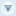Subscribe to our news:PartnersCustomers

## OnCalculateFieldsThis event occurs whenever the values of calculated columns are (re)computed in read-only views (List, Print, Export, etc). Live examples.

Signature:

function OnCalculateFields(\$rowData, &fieldName, &\$value)

Parameters:

 \$rowData An associative array of values of the currently processed row \$fieldName The name of the currently processed calculated column \$value The value to be assigned to the column

Example 1:

This code snippet sets the value of the full_name column according to the values stored in the first_name and last_name columns.

if (\$fieldName == 'full_name') {

\$value = \$rowData['first_name'] . ' ' . \$rowData['last_name'];

Example 2:

This code snippet sets the value of the age column according to the birthday.

if (\$fieldName == 'age') {

\$dateOfBirth = new DateTime(\$rowData['birthday']);

\$dateInterval = \$dateOfBirth->diff(new DateTime());

\$value = \$dateInterval->format('%y');

}